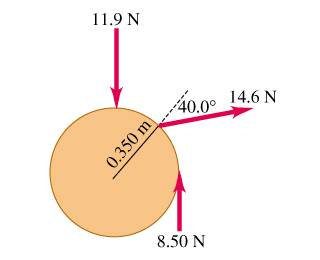# Net Torque magnitude and direction

## Homework Statement

Three forces are applied to a wheel of radius 0.350m, as shown in the figure. One force is perpendicular to the rim, one is tangent to it, and the other one makes a 40.0 angle with the radius.a) What is the magnitude of the net torque on the wheel due to these three forces for an axis perpendicular to the wheel and passing through its center?

b) What is the direction of the net torque in part (A).

## The Attempt at a Solution

for the one that is perpendicular

tor = 11.9 sin 90 * .350 = 4.165

for the one that is at an angle

tor = 14.6 sin 40 * .350 = 3.28

or the one tangent

tor = 8.50 sin 90 *.350 = 2.98

4.165+3.28+2.98 = 10.42?

Shooting Star
Homework Helper
The perp force passes through the centre. How can it have a torque about the centre? It should be sin 0, not sin 90.

Also notice that torque due to the tangential force is opposite to the torque due to the force at an angle. You have to subtract, not add.

G01
Homework Helper
Gold Member
Remember where the angle is measured from in these problems. The angle you are talking about is the angle between the radius vector and the force vector. So, in the case of the perpendicular force, this means that the angle is 0 degrees, since the force is parallel to the radial direction.

Other than this, the only problem is your signs. The two other torques cause the wheel to rotate in opposite directions, so the should have opposite signs. Which one should be negative depends on how you have positive and negative behind. The standard is to say that counterclockwise torques are positive. So, knowing this, can you give me the correct signs for the torques?

for the one that is perpendicular

tor = 11.9 sin 0 * .350 = 0

for the one that is at an angle

tor = -14.6 sin 40 * .350 = -3.28

or the one tangent

tor = 8.50 sin 90 *.350 = 2.98

0 - 3.28 + 2.98 = -.3096

so it will be turning to the right..(if I used the standard).

so it will be going out of the page?

Last edited:
Shooting Star
Homework Helper
I assume that this is happening where gravity is zero. In that case, the wheel need not rotate out of the page. You have to see how the CM of the body moves. When you wrote that it’ll be rolling out of the page, you have perhaps considered the wheel to be standing on a horizontal plane, with gravity.

If the centre of the wheel is fixed, it cannot move. Otherwise, If these forces continue to act on the body in exactly this way, then the body will be in translation and rotating motion. The resultant of all the forces, including the perp one, will accelerate the body. The CM will move as if the resultant is acting on the total mass sitting there. At the same time, the body will have angular acceleration, and rotate faster and faster. Which way the body moves will depend only on the translatory motion, which you can easily calculate.

ummmmm....I got lost in your explanation.

So the signs of the torques do no help me decide in which way its moving?

I thought it was going to be that way :(

Shooting Star
Homework Helper
ummmmm....I got lost in your explanation.

So the signs of the torques do no help me decide in which way its moving?

I thought it was going to be that way :(

I have assumed no gravity or other forces except the ones shown.

Suppose there are two tangential forces acting on diametrically opposite points of the wheel, equal and opposite, and no other force. Both of them produce torques in the same direction, say clockwise. Then the net torque is clockwise. Do you thing the wheel will roll to the right out of the page? No, it will simply rotate about the centre.

The wheel won’t move as a whole since the total force is zero, and the CM will stay put, because initial velocity was zero. So, it’s not the net torque that determines the direction of motion. It’s the resultant of the forces and the initial velocity of the CM. The net torque determines how fast it rotates wrt the CM frame.

(If the wheel is resting on horizontal plane, then the picture changes because there are other forces acting on it.)

There’s a basic theorem in mechanics which states that the motion of the CM of a body is as if the total mass placed on the CM is being acted on by the resultant of the forces and the torque of the resultant force about the CM is the sum of all the torques due to these forces about the CM.